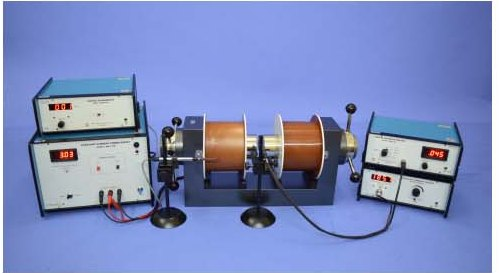# Viva Questions for HALL Effect1. How is Hall’s coefficient related with carrier concentration?
2. On what factors does the sign of the Hall’s coefficient depend?
3. What is the sign of Hall Coefficient for an intrinsic semiconductor?
4. Is it possible to measure Hall’s coefficient for metals?
5. Why is the Hall coefficient of semiconductors many orders of magnitude sgreater than
that of metals?
6. How is Hall’s coefficient related to the Hall’s Voltage (VH) and the thickness(t)? Why
should the sample be thin?
7. In what units is Hall’s coefficient measured in?
8. De ne carrier concentration, electrical conductivity, and mobility of charge carriers.
How are they related?
9. What are some of the requirements for a sample to be used for the determination of Hall’s Coefficient?
10. Explain the increase of the resistance of the sample with the increase in magnetic field.
11. Define hall coefficient.
12. How does mobility depend on electrical conductivity?
13. Define Hall angle.
14. Which type of charge has greater mobility?
15. What is the eff ect of temperature on Hall coefficient of a lightly doped semiconductor?
16. Do the holes actually move?
17. Does the Hall coefficient depend on the dimensions of the sample?## 3 thoughts on “Viva Questions for HALL Effect”

1. Thanks dear they are very useful…

1. Thanks for your comment. People like you keep me going. 🙂

2. can you provide answer for these questions.
Good collection of question…